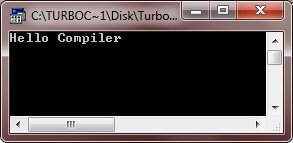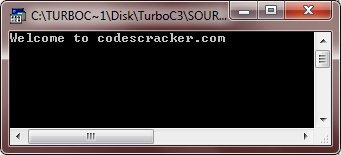# C Basic Syntax

Basic syntax of a simple C program contains header files, main() function and then program code. Here is the basic syntax of a simple and smallest C program:

```header files
return_type main()
{
program codes
}```

Every C program must contains a function called main(), because program execution starts from main(). For example, here is the simple and smallest C program, which would print "Hello Compiler" on the output screen:

```#include<stdio.h>
#include<conio.h>
void main()
{
clrscr();
printf("Hello Compiler");
getch();
}```

Here is the sample output of the above C program:From the above program, you can filter out like this:

```#include<stdio.h>
#include<conio.h>```

denotes to

`header files`

and

`void main()`

denotes to

`return_type main()`

and then

```clrscr();
printf("Hello Compiler");
getch();```

denotes to

`program code`

## Tokens in C

In C, a token is either a keyword or a constant or a string constant or a symbol or an identifier. And a C program consists of various tokens, depends on, how long the program is. Let's look at the following example, here the statement consists of total five tokens:

`printf("Hello Compiler");`

Here, the individual tokens from the above statements are:

```printf
(
"Hello Compiler"
)
;```

## Semicolons in C

In C programming, a semicolon is used to terminate a statement. Semicolon in C also called as statement terminator because each individual statement must be ended with a statement terminator or semicolon. This tells the compiler that this statement is completed. And it indicates that the end of one logical entity or statements. If semicolon is not placed at the end of any statements, then the program will not compile, generates error message on compilation. Let's look at the following example, here there are two different statements terminated by the semicolon each:

```printf("Hello Compiler");
getch();```

In C Programming, comments are just like a helping text in your C program. They are ignored by the compiler. Comments are very useful in reviewing the code. Since at the time of reviewing the code, given comments helps a lot for a programmer. As comments, programmer can add useful information or reminder about some line of code or something for other purposes.

In C language, there are the following two types of comments:

• Single-line comments - starts from // to the end of line
• Multi-line comments - starts from /* and ends with */

Here is an example program, demonstrates both, the single-line comments and the multi-line comments:

```/* C Basic Syntax - This program demonstrates
* This is a multi-line comments
*/

#include<stdio.h>
#include<conio.h>
void main()
{
clrscr();  // to clear the screen. This is a single-line comment

printf("Welcome to codescracker.com");

/* The above statement prints
* Welcome to codescracker.com
* on the output screen. And this is
*/

/* This is another multi-line comment.
As you can see, from the output screen
all the comments are ignored by the compiler */

getch();   // holds output screen until user press a key
}```

Here is the sample output of this C program. You will see, only "Welcome to codescracker.com" will be printed on the output screen. And as already told, all the comments are ignored by the compiler. Because comments can only be read by the programmer.## Identifiers in C

In C programming, an identifier is the name that is used to identify a variables, functions, or any other user-defined terms. An identifier can start with letters (A to Z) or (a to z) or an underscore ( _ ) followed by zero or more letters, underscores, and digits (0 to 9)

C does not allows punctuation characters (special characters) like @, \$, and % within identifiers. Since C programming is a case sensitive programming language. Thus, Total and total are the two different identifiers in C. Here the following table lists some valid identifiers :

 sum total avg perc _cal str c_523 angelo23 _lee find_sum c23g5 num res cal calculate_area

Here is an example program. Just concentrate on this. This program also illustrates that the num and Num or name and Name are different-different identifiers:

```#include<stdio.h>
#include<conio.h>
void main()
{
int num, Num;
char name, Name;
clrscr();

printf("Enter the two number: ");
scanf("%d%d", &num, &Num);
printf("\nYou entered:\n");
printf("%d\t%d\n\n", num, Num);

fflush(stdin);
printf("\nEnter your two friends name: ");
gets(name);
gets(Name);
printf("%s and %s", name, Name);

getch();
}```

Here is the sample run of the above C program:## C Keywords

Keywords are the reserved words having special meaning. Here the following table lists the C keywords:

 auto double int struct break else switch long case enum register typedef extern char return union const float short unsigned continue for signed void default sizeof goto volatile do if static while

## Whitespaces in C

In C programming, any line containing only whitespace, like with a comment, is known as a blank line, and the C compiler completely ignores it. Whitespace is a term used in C programming that describe the blanks, tabs, newline characters and the comments. Whitespace separates one part of a statement from another part of a statement and it enables the compiler to identify that where one element in a statement, like int, ends and the next element begins. Here this statement:

`int sum;`

There must be at least one whitespace character (generally a space) between int (data type) and sum (variable) for a compiler to be able to distinguish them. If you will not insert a whitespace between them then it will become intsum and will be treated as an identifier.

### Examples

Here are the list of some examples that you can go for:

Tools
Calculator